Get inspired by the success stories of our students in IIT JAM MS, ISI  MStat, CMI MSc DS.  Learn More

# Trace & Determinant | ISI MStat 2017 Problem 1 | PSBThis is a beautiful problem from ISI MStat 2017 PSB Problem 1 based on matrices. We provide a detailed solution with the prerequisites mentioned explicitly.

## Problem - ISI MStat 2017 Problem 1

Let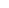and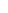be real numbers. Show that there exists a unique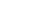real symmetric matrix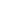with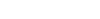and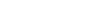if and only if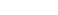.

## Prerequisites

• Trace
• Determinant
• Sridhar Acharya Formula

## Solution

Let ,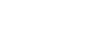be a uniquereal symmetric matrix , with x,y,z belongs to real .

Given , trace(A)=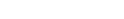----(1)and

Det(A)=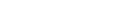----(2)

1st solution :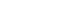from (1) putting this in (2) we get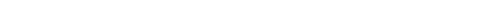Now using , Sridhar Acharya Formula we get ,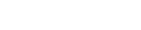Now as given , A is unique so y can't take two different values hence this part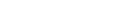must be zero

i.e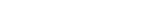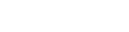Again , as A is unique matrix z can't take two different values . Hence z must be equal to zero

i.e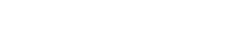( Hence proved)

2nd solution : See if we interchange x by y then all the properties of A remains same i.e trace and determinant . But it can't be possible as we assume that A is unique hence x and y must be equal i.e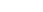.

From (1) we get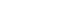Again if we interchange z by -z then all the properties of A remains same i.e trace and determinant . But it can't be possible as we assume that A is unique hence z and -z must be equal i.e.

From (2) we get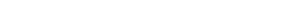(Hence proved )

Now , we will assume that, where a and b are real and show that the matrix is unique .

Let ,be areal symmetric matrix with x,y,z belongs to real

Given trace(A)=---(3)

and Det(A)=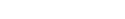---(4)

Another thing is given thatSo using (3) and (4) in (5) we get ,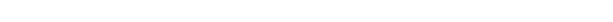i.e sum of two squares is equal to 0 which implies individual squares are equal to zero .

Hence ,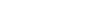and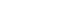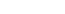and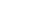which giveandHence ,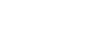is unique ( proved )

we have proved both if and only if part . Hence we are done!

This is a beautiful problem from ISI MStat 2017 PSB Problem 1 based on matrices. We provide a detailed solution with the prerequisites mentioned explicitly.

## Problem - ISI MStat 2017 Problem 1

Letandbe real numbers. Show that there exists a uniquereal symmetric matrixwithandif and only if.

## Prerequisites

• Trace
• Determinant
• Sridhar Acharya Formula

## Solution

Let ,be a uniquereal symmetric matrix , with x,y,z belongs to real .

Given , trace(A)=----(1)and

Det(A)=----(2)

1st solution :from (1) putting this in (2) we getNow using , Sridhar Acharya Formula we get ,Now as given , A is unique so y can't take two different values hence this partmust be zero

i.eAgain , as A is unique matrix z can't take two different values . Hence z must be equal to zero

i.e( Hence proved)

2nd solution : See if we interchange x by y then all the properties of A remains same i.e trace and determinant . But it can't be possible as we assume that A is unique hence x and y must be equal i.e.

From (1) we getAgain if we interchange z by -z then all the properties of A remains same i.e trace and determinant . But it can't be possible as we assume that A is unique hence z and -z must be equal i.e.

From (2) we get(Hence proved )

Now , we will assume that, where a and b are real and show that the matrix is unique .

Let ,be areal symmetric matrix with x,y,z belongs to real

Given trace(A)=---(3)

and Det(A)=---(4)

Another thing is given thatSo using (3) and (4) in (5) we get ,i.e sum of two squares is equal to 0 which implies individual squares are equal to zero .

Hence ,andandwhich giveandHence ,is unique ( proved )

we have proved both if and only if part . Hence we are done!

This site uses Akismet to reduce spam. Learn how your comment data is processed.

### Knowledge Partner